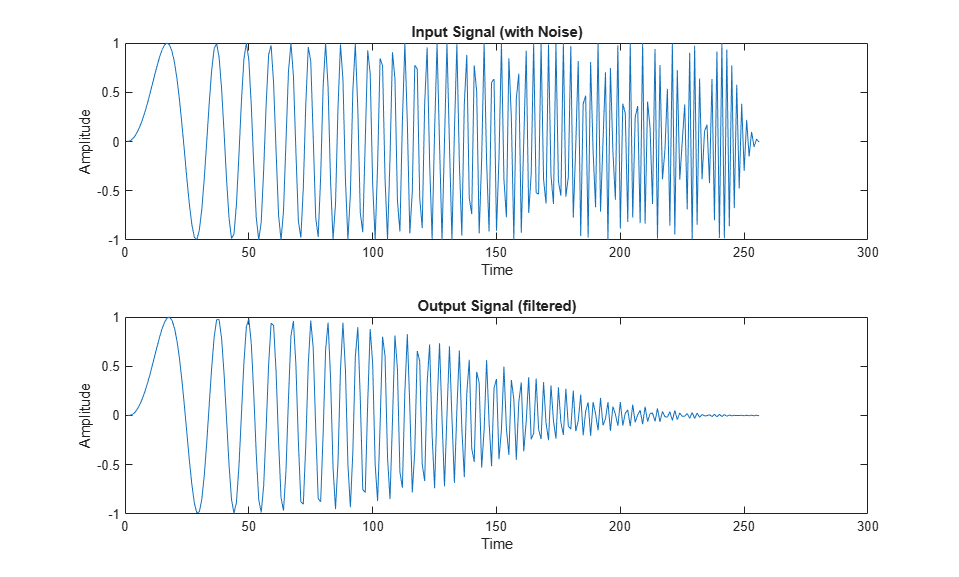# SystemC Code Generation for DF2T Filter

You can generate SystemC code from MATLAB® design for direct-form II transposed filter.

### MATLAB Design

Set up the `df2t_filter` model and test bench for this example.

```mlhdlc_demo_setup('df2t_filter'); % Design Sqrt design_name = 'mlhdlc_df2t_filter'; % Test Bench for Sqrt testbench_name = 'mlhdlc_df2t_filter_tb'; ```

Review the `df2t_filter` design

```dbtype(design_name) ```
```1 %#codegen 2 function y = mlhdlc_df2t_filter(x) 3 4 % Copyright 2011-2015 The MathWorks, Inc. 5 6 persistent z; 7 if isempty(z) 8 % Filter states as a column vector 9 z = zeros(2,1); 10 end 11 12 % Filter coefficients as constants 13 b = [0.29290771484375 0.585784912109375 0.292907714843750]; 14 a = [1.0 0.0 0.171600341796875]; 15 16 y = b(1)*x + z(1); 17 z(1) = (b(2)*x + z(2)) - a(2) * y; 18 z(2) = b(3)*x - a(3) * y; 19 20 end ```

### Simulate the Design

It is a best practice to simulate the design with the test bench prior to code generation to check for run-time errors.

```mlhdlc_df2t_filter_tb ```### Create a HDL Coder™ Project

Create an HDL Coder project.

```coder -hdlcoder -new mlhdlc_df2t_prj ```

Next, add the file `mlhdlc_df2t_filter.m` to the project as the MATLAB Function and `mlhdlc_df2t_filter_tb.m` as the MATLAB Test Bench.

### Run Fixed-Point Conversion and SystemC Code Generation

To generate SystemC code from the MATLAB design:

1. At the MATLAB command line, set up the path for SystemC code generation by using the function `hdlsetuphlstoolpath`.